# 二叉树链式结构的实现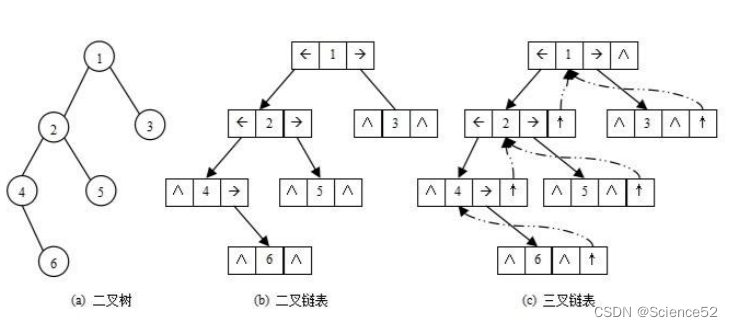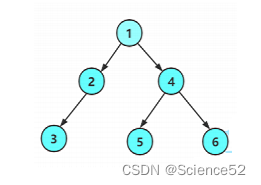``````//手动创造出一颗二叉树
BTNode* BinaryTreeCreate()
{
BTNode* n1 = (BTNode*)malloc(sizeof(BTNode));
assert(n1);
BTNode* n2 = (BTNode*)malloc(sizeof(BTNode));
assert(n2);
BTNode* n3 = (BTNode*)malloc(sizeof(BTNode));
assert(n3);
BTNode* n4 = (BTNode*)malloc(sizeof(BTNode));
assert(n4);
BTNode* n5 = (BTNode*)malloc(sizeof(BTNode));
assert(n5);
BTNode* n6 = (BTNode*)malloc(sizeof(BTNode));
assert(n6);
//给节点赋值
n1->data = 1;
n2->data = 2;
n3->data = 3;
n4->data = 4;
n5->data = 5;
n6->data = 6;

//建立链接关系
n1->left = n2;
n1->right = n4;
n2->left = n3;
n2->right = NULL;
n3->left = NULL;
n3->right = NULL;
n4->left = n5;
n4->right = n6;
n5->left = NULL;
n5->right = NULL;
n6->left = NULL;
n6->right = NULL;
return n1;
}``````

二叉树的遍历：(前序、中序以及后序遍历）

1. 前序遍历(Preorder Traversal 亦称先序遍历)——访问根结点的操作发生在遍历其左右子树之前。

2. 中序遍历(Inorder Traversal)——访问根结点的操作发生在遍历其左右子树之中（间）。

3. 后序遍历(Postorder Traversal)——访问根结点的操作发生在遍历其左右子树之后。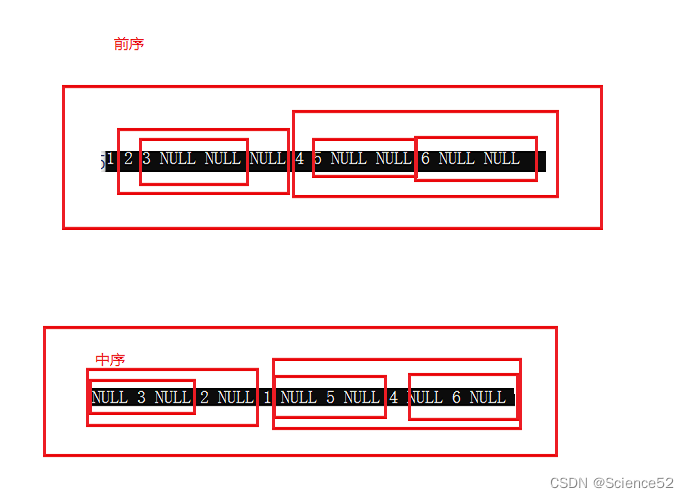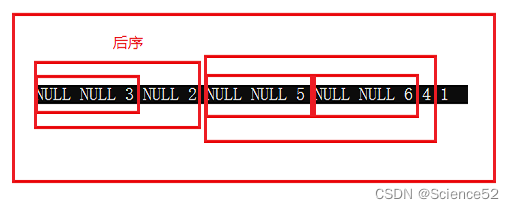``````// 二叉树前序遍历
void BinaryTreePrevOrder(BTNode* root)///根 左子树 右子树
{
if (root == NULL)
{
printf("NULL ");
return;
}
printf("%d ", root->data);
BinaryTreePrevOrder(root->left);
BinaryTreePrevOrder(root->right);
}
// 二叉树中序遍历
void BinaryTreeInOrder(BTNode* root)//左子树  根  右子树
{
if (root == NULL)
{
printf("NULL ");
return;
}
BinaryTreeInOrder(root->left);
printf("%d ", root->data);
BinaryTreeInOrder(root->right);
}
// 二叉树后序遍历
void BinaryTreePostOrder(BTNode* root)//左子树  右子树  根
{
if (root == NULL)
{
printf("NULL ");
return;
}
BinaryTreePostOrder(root->left);
BinaryTreePostOrder(root->right);
printf("%d ", root->data);
}
``````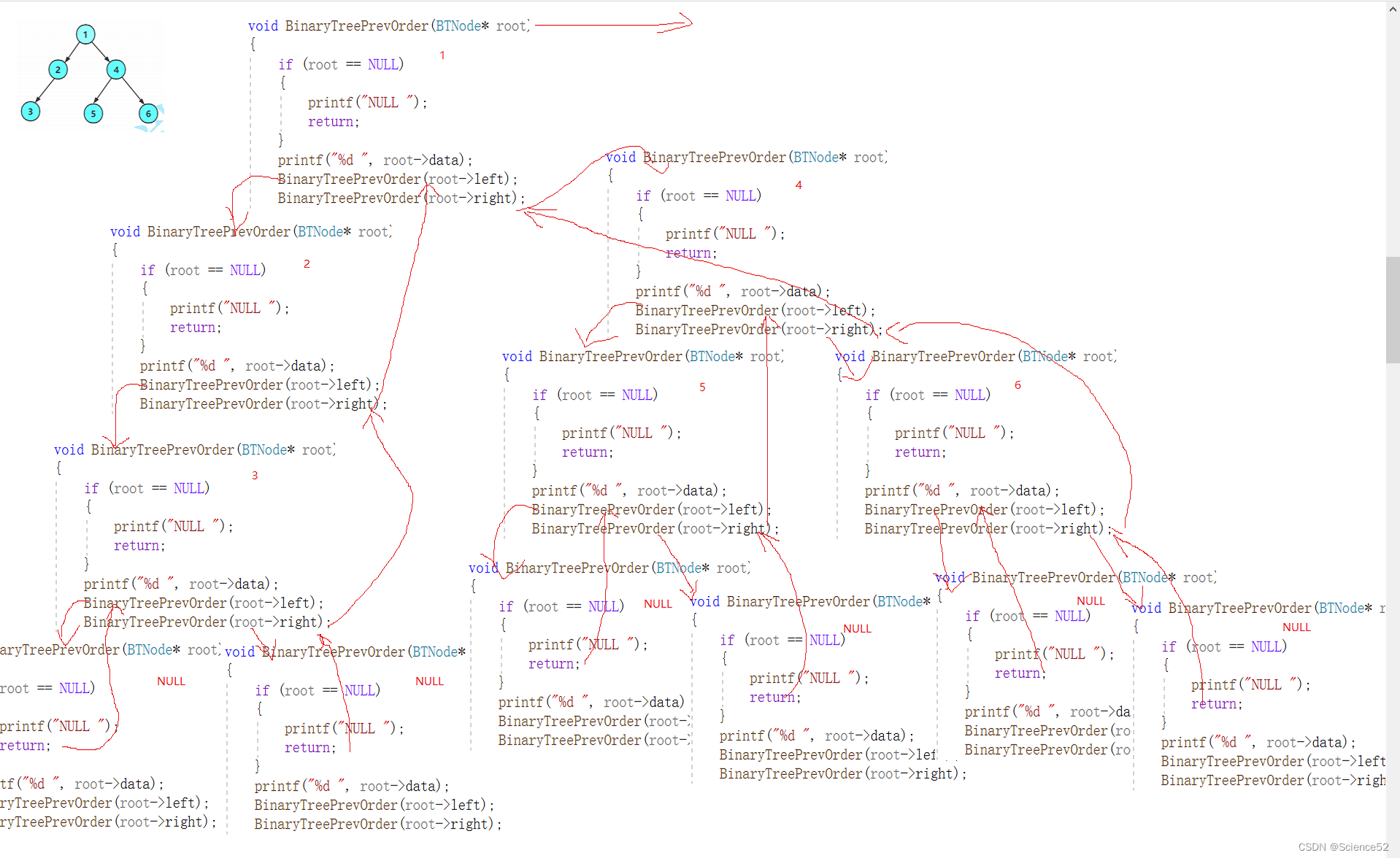二叉树的其他接口：

``````// 二叉树节点个数
int BinaryTreeSize(BTNode* root)
{
if (root == NULL)
{
return 0;
}
//返回左边的和右边的还有自己
return BinaryTreeSize(root->left) + BinaryTreeSize(root->right) + 1;
}

// 二叉树叶子节点个数
int BinaryTreeLeafSize(BTNode* root)
{
if (root == NULL)
{
return 0;
}
//判断叶子节点
if (root->left == NULL && root->right == NULL)
{
return 1;
}
return BinaryTreeLeafSize(root->left) + BinaryTreeLeafSize(root->right);
}

// 二叉树第k层节点个数
int BinaryTreeLevelKSize(BTNode* root, int k)
{
assert(k > 0);
if (root == NULL)
{
return 0;
}
if (k == 1)
{
return 1;
}
return BinaryTreeLevelKSize(root->left, k-1) + BinaryTreeLevelKSize(root->right, k-1);
}

// 二叉树查找值为x的节点
BTNode* BinaryTreeFind(BTNode* root, BTDataType x)
{
if (root == NULL)
{
return NULL;
}
if (root->data == x)
{
return root;
}
//看根的左子树有没有
BTNode* left = BinaryTreeFind(root->left, x);
if (left)
return left;
//看根的右子树有没有
BTNode* right = BinaryTreeFind(root->right, x);
if (right)
return right;
//都没有
return NULL;
}

//二叉树的高度
int TreeHeight(BTNode* root)
{
//左子树和右子树的和加根自己也就是加1就是结果
if (root == NULL)
{
return 0;
}
int leftHeight = TreeHeight(root->left);
int rightHeight = TreeHeight(root->right);
return leftHeight > rightHeight ? leftHeight + 1 : rightHeight + 1;
}

// 通过前序遍历的数组"ABD##E#H##CF##G##"构建二叉树
BTNode* BinaryTreeCreateByPrev(BTDataType* a, int* pi)
{
//遵守根左子树右子树的规则，从最后一层开始链接起来
if (a[*pi] == '#')
{
(*pi)++;
return NULL;
}
BTNode* root = (BTNode*)malloc(sizeof(BTNode));
if (root == NULL)
{
perror("malloc fail");
return NULL;
}
root->data = a[(*pi)++];
//链接根的左右
root->left = BinaryTreeCreateByPrev(a, pi);
root->right = BinaryTreeCreateByPrev(a, pi);
return root;
}

//二叉树的销毁
void BinaryTreeDestory(BTNode* root)
{
//递归到最后一个再不断向上销毁
if (root == NULL)
{
return;
}
BinaryTreeDestory(root->left);
BinaryTreeDestory(root->right);
free(root);
}``````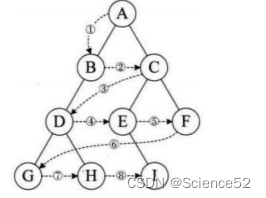``````// 层序遍历
void BinaryTreeLevelOrder(BTNode* root)
{
//利用队列的性质，先将根的左右子树进队，然后每次出队的时候都进出队的那个元素的两个子树
Qe q;
QueueInit(&q);
//先讲根进队列
QueuePush(&q,root);
while (!QueueEmpty(&q))
{
BTNode* front = QueueFront(&q);
printf("%d ", front->data);
QueuePop(&q);
//出一个根进根的左右子树
if (front->left)
{
QueuePush(&q, front->left);
}
if (front->right)
{
QueuePush(&q, front->right);
}
}
}
``````

接下来就是和层序遍历的思想很像的判断一棵树是否为完全二叉树：主要思路也是利用队列来实现，和层序遍历相似，把全部元素入队列，如果遇到空指针，并且空指针的后面还有其他元素则说明这不是完全二叉树，否则就是完全二叉树，下面我们用代码来实现：

``````// 判断二叉树是否是完全二叉树
int BinaryTreeComplete(BTNode* root)
{
//利用层序遍历的思想，如果最后出到空之后的元素中还存在非空元素，就不是完全二叉树
Qe q;
QueueInit(&q);
//先讲根进队列
QueuePush(&q, root);
while (!QueueEmpty(&q))
{
BTNode* front = QueueFront(&q);
QueuePop(&q);
//出到空指针就跳出去
if (front == NULL)
{
break;
}
//出一个根进根的左右子树,这里空也要进去，因为后面要判断
QueuePush(&q, front->left);
QueuePush(&q, front->right);
}
//到这里所有元素都进入了并且如果是完全二叉树的话就只剩空
while (!QueueEmpty(&q))
{
BTNode* front = QueueFront(&q);
QueuePop(&q);
if (front != NULL)
{
QueueDestroy(&q);
return false;
}
}
QueueDestroy(&q);
return true;
}
``````